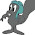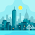## Sunday, February 17, 2013

### A look at strucchange and segmented

After last week's post it was commented that strucchange and segmented would be more suitable for my purpose. I had a look at both. Strucchange can find a jump in a time series, which was what I was looking for. In contrast segmented is more suitable for occasions where rates of effect. This made me think of a post on climate change I made earlier, so I applied segmented to those data

### Strucchange and Nile data

Strucchange is a package to detect jumps in data. They have an example of the effect of the Aswan dam on Nile water. Those data are suitable to compare the methods available. For strucchange this is just following the example. The result is an effect on water flow around 1898. There is just one catch. It needs a time series, so regular data over time.
library(strucchange)
library(segmented)
library(R2jags)
library(tree)

data("Nile")
plot(Nile)
bp.nile <- breakpoints(Nile ~ 1)
ci.nile <- confint(bp.nile, breaks = 1)
lines(ci.nile)
Interestingly tree gives 1898.5, about the same answer. Segmented does not work. It is not devised for this kind of step changes.
NileDF <- data.frame(Nile=as.numeric(Nile),year=1871:1970)
tree(Nile ~ year,data=NileDF)

node), split, n, deviance, yval
* denotes terminal node

1) root 100 2835000  919.4

2) year < 1898.5 28  492000 1098.0
4) year < 1889.5 19  388200 1067.0
8) year < 1880.5 10  205200 1133.0 *
9) year > 1880.5 9   92680  994.6 *
5) year > 1889.5 9   48760 1162.0 *
3) year > 1898.5 72 1105000  850.0
6) year < 1953.5 55  802300  836.1 *
7) year > 1953.5 17  258600  894.7
14) year < 1965.5 12  114300  947.8 *
15) year > 1965.5 5   29480  767.4 *

segmented(lm(Nile ~1,data=NileDF),~ year,psi=list(year=c(1890)))

Error in segmented.lm(lm(Nile ~ 1, data = NileDF), ~year,
psi = list(year = c(1900))) :
only 1 datum in an interval: breakpoint(s) at the boundary or too close each other

The Bayes model is working the Nile data, but the result is not quite the same as strucchange. The interval for the dates is a bit smaller.
model1 <- function() {
for ( i in 1:N ) {
category[i] <- (xx[i]>limit) + 1
yy[i] ~ dnorm( mu[category[i]] , tau )
}
tau <- 1/pow(sigma,2)
sigma ~ dunif(0,100)
mu ~ dnorm(0,1E-6)
mu ~ dnorm(0,1E-6)
limit ~ dbeta(1,1)
}
datain <- list(yy=c(NileDF\$Nile),xx=seq(0,1,length.out=length(Nile)),N=length(Nile))
params <- c('mu','sigma','limit')
inits <- function() {
list(mu = rnorm(2,0,1),
sigma = runif(1,0,1),
limit=runif(1,0,1))
}
jagsfit <- jags(datain, model=model1, inits=inits, parameters=params,
progress.bar="gui",n.iter=10000)
limit = as.numeric(jagsfit\$BUGSoutput\$sims.array[,,'limit'])*100+1871
png('16feb13.2.png')
plot(density(limit),main='Nile flooding',xlab='Year')

### Segmented and the climate data

As written above, I used these climate data before. I now realize they are updated every month, 2012 is now complete. As I want to use these data again, a few lines to process the data prior to read.table are added. My choice was to use three breaks, approximately near 1900, 1940 and 1970, to initiate the algorithm. The data shows four phases. A decrease till 1910, an increase till 1940, a flat till 1970 then an increase.
# data from http://data.giss.nasa.gov/gistemp/tabledata_v3/GLB.Ts+dSST.txt
#remove empty lines, print notes, then remove them
Datain <- Datain[grep('^ *\$',Datain,invert=TRUE)]
grep('^(Year|[[:digit:]]{4})',Datain,value=TRUE,invert=TRUE)
 " GLOBAL Land-Ocean Temperature Index in 0.01 degrees Celsius base period: 1951-1980"
 " sources: GHCN-v3 1880-12/2012 + SST: ERSST 1880-12/2012"
 " using elimination of outliers and homogeneity adjustment"
 " Notes: 1950 DJF = Dec 1949 - Feb 1950 ; ***** = missing"
 " AnnMean"
 "Divide by 100 to get changes in degrees Celsius (deg-C)."
 "Multiply that result by 1.8(=9/5) to get changes in degrees Fahrenheit (deg-F)."
 "Best estimate for absolute global mean for 1951-1980 is 14.0 deg-C or 57.2 deg-F,"
 "so add that to the temperature change if you want to use an absolute scale"
 "(this note applies to global annual means only, J-D and D-N !)"
 "Example -- Table Value : 40"
 " change : 0.40 deg-C or 0.72 deg-F"
 "abs. scale if global annual mean : 14.40 deg-C or 57.92 deg-F"

Datain <- Datain[grep('^(Year|[[:digit:]]{4})',Datain)]
#column header is repeated. remove all but first
header <- which(Datain ==Datain)
# and fix *** for missing - the ' ' separator is missing D-N 1880
Datain <- gsub('\\*+',' NA',Datain)
plot(J.D ~ Year,data=r1,type='l',,main='GLOBAL Land-Ocean Temperature Index',ylab='0.01 degrees C base period: 1951-1980')
seg <- segmented(lm(J.D~Year,data=r1),~ Year, list(Year=c(1900,1940,1970)))
lines(seg,col='red')

slope(seg)
\$Year
Est. St.Err. t value CI(95%).l CI(95%).u
slope1 -0.68530  0.1647 -4.1600   -1.0110   -0.3593
slope2  1.29300  0.2013  6.4220    0.8944    1.6910
slope3 -0.04426  0.1728 -0.2562   -0.3862    0.2977
slope4  1.67400  0.1095 15.2900    1.4580    1.8910

#### Note

Given the sensitivity of climate I feel obliged to mention I am not a climate specialist and hence cannot vouch for the appropriateness of the segmented model for these climate data.

1.Hmmm, I get

> ci.nile <- confint(bp.nile, breaks = 1)
Error in FUN(c(15, 16, 17, 18, 19, 20, 21, 22, 23, 24, 25, 26, 27, 28, :
could not find function "RSS"

Does this ring any bells? Happy to provide more details if not.

1.That part is a direct copy of the example, so it is a bit strange it doesn't work

2.This comment has been removed by the author.

3.Here, how to find the sensitivity of that model using breaking points? how to do adjust the breaking points?